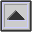PARTICLE VELOCITY

The particles or molecules of a medium are displaced from their random motion in the presence of a SOUND WAVE, and are set into OSCILLATION by the frequency associated with the wave. The speed of the particle during displacement is called the particle velocity, and its relation to the SOUND PRESSURE p is given by the following relation:

p = r . c . u

where r is the density of the medium, c is the SPEED OF SOUND, and u is the particle velocity which is the ROOT MEAN SQUARE of the instantaneous particle velocities over a time interval at the given position. The AMPLITUDE of the displacement d is related to the particle velocity by the equation:

d = u / 2pf

where f is the frequency of the wave. These displacements are very small, being, for example, of the order of one-millionth of an inch in the case of normal conversation at 10 feet.

home